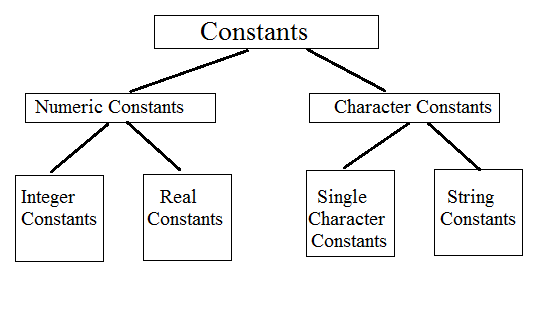# Constants :

Constants in C refer to fixed values that do not change during the execution of a program, i.e once the value is declared, it is not changed throughout the program. C supports several types of constants as shown in he figureThe following are the four basic types of constants.

• Integer Constants
• Real Constants
• Single Character Constants
• String Constants

# Integer Constants

An Integer constant refers to sequence of digits with out decimal point.

### Rules for constructing integer constants :

• An integer constant must have at least one digit.
• It must not have a decimal point.
• It could be either positive or negative.
• If no sign precedes an integer constant, it is assumed to be positive.
• No commas or blanks are allowed within an integer constant.
• The allowable range for integer constants is -32768 to 32767 for 16 bit machine.

An integer constant can be represented in any one of the following three number systems.

1. Decimal Integers
2. Octal Integers

### 1. Decimal Integers :

They consists of a set of digits, 0 through 9 without decimal point or fractional part, preceded by an optional - or + sign. Embedded spaces, commmas, and non-digit characters are not permitted between digits. They cannot exceed minimum and maximum bounds.

Valid decimal integers : 123, -321, 0, 654321, +78 etc.
Invalid decimal integers : 15 750, 20.000, \$1000 etc.

### 2. Octal Integers :

They consists of any combination of digits from the set 0 through 7, with a leading 0.

Example : 037, 0, 0435, 0551 etc.

### 3. Octal Integers :

A sequence of digits preceded by 0x or 0X is considered as hexadecimal integer. They may also include alphabets A through F or a through f.

A through F represent the numbers 10 through 15.

Examples : 0X2, 0X9F, 0xbcd, 0x etc.

The largest integer value that can be stored is machine dependent. It is 32767 on 16 bit machines and 2147483647 on 32 bit machines. It is also possible to store larger integer constants on these machines by appending qualifiers such as U, L and UL to the constants.

Example :
 56789U or 56789u (Unsigned integer) 987612347UL or 987612347ul (Unsigned long integer) 9876543L or 9876543l (Long integer)

# Real Constants :

Integer numbers are inadequate to represent quantities that vary continuously, such as distances, heights, temperatures, prices and so on. In such cases real constants are used.

The real constants could be written in following two forms,

• fractional (decimal) form
• exponential (scientific) form

### Fractional Form :

These quantities are represented by numbers containing fractional part like 17.548. Such numbers are called fractional form of real constants.

Rules to construct real constants which are expressed in fractional form

1. A real constant must have atleast one digit.
2. It must have a decimal point
3. It could be either positive or negative
4. Default sign is positive
5. No commas or blanks are allowed within real constants
6. It is possible to omit digits before the decimal point or digits after the decimal point.
Example's :
0.0083, -0.75, 435.36, +247.0
215., .95, -.71, +5

### Exponential Form :

In exponential form of representation, the real constant is represented in two parts. The part appearing before 'e' is called mantissa, whereas the part following 'e' is called exponent.

The general form is mantissa e exponent

The mantissa is either a real number expressed in decimal notation or an integer.

Rules to construct real constants which are expressed in exponential form

• Default sign of mantissa part is positive
• The exponent must have at least one digit, which must be a positive or negative integer. Default sign is positive.
• The letter 'e' separating the mantissa and the exponent can be written in either lowercase or uppercase.
• Range of real constants expressed in exponential form is -3.4e38 to 3.4e38
Examples : 0.65 e 4, 12 e -2, 1.5 e +5, 3.18 E 3, -1.2E-1

Exponential notation is useful for representing numbers that are either very large very small in magnitude. For example 7500000000 may be written as 7.5E9 or 75E8.
Simillarly, -0.000000363 is equivalent to -3.68E-7.

# Single Character Constants :

A single character constant (or simply character constant) contains a single character enclosed with in a pair of single quote marks.

Examples : '5', 'X', ';', ''
• Character constant have integer values known as ASCII values the statement printf("%d",'a'); would print the number 97, the ASCII value of the character 'a'.
Similarly the statement printf("%c",97); would output the character 'a'.
• Each Character constant represents an integer value, so it is also possible to perform arithmetic operations on character constrants.
• Maximum of one character is taken within a character constant.

# String Constants :

A string constant is a sequence of characters enclosed in double quotes. The characters may be letters, numbers, special characters and blank spaces.

Examples : "hello", "1987", "WELL DONE", "?....!", "5+3", "X"
• A single character string constant does not have an equivalent integer value while a character constant has an integer value.
• String constants can not be used in arithmetic operations
• Character strings are often used in programs to build meaningful programs.

### Backslash character constants :

C supports some backslash character constants that are used in output functions.
Each character constant has its own purpose and contains two characters. These character combinations are known as escape sequences.

### Backslash Character Constants :

Backslash Character Backslash Character
Constant Meaning Constant Meaning
'\a' Audible alert(bell) '\v' vertical tab
'\b' back space '\'' single quote
'\f' form feed '\"' double quote
'\n' new line '\?' question mark
'\r' carriage return '\\' backslash
'\t' horizontal line '\0' Null
##### 0 Komentar untuk "Constants and Basic types of Constants"